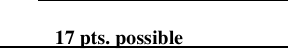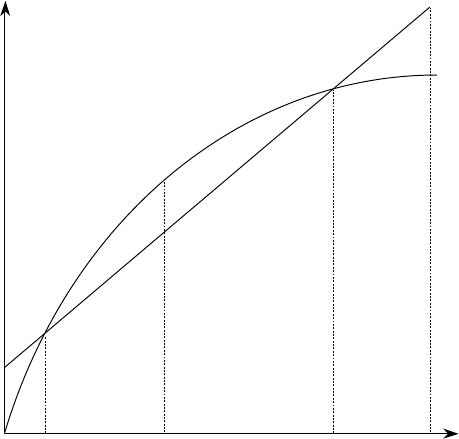Study Guides (400,000)
US (230,000)
UH (60)
PHYS (30)
All (30)
Midterm

# Hawaii PHYS 151 exam151m1-05sprExam

Department
Physics
Course Code
PHYS 151
Professor
All
Study Guide
Midterm

This preview shows pages 1-2. to view the full 6 pages of the document.Physics 151 Roster No.:
Score: 17 pts. possible
SPRING 2005 Midterm Exam #1, Part A
Exam time limit: 50 minutes. You may use a calculator and both sides of ONE sheet of notes, handwritten
only. Closed book; no collaboration. For multiple choice questions, circle the letter of the one best answer (unless
g = 9.80 m/s2
1. (1 pt.) The physical quantity R = 6.400 × 106 m has how many significant figures?
A. 2 D. 5
B. 3 E. 6
C. 4 F. 7
2. (2 pts.) Your friend has 850 music files on her computer, each of which has an average size of 3.0 Mb (b = byte).
She wants to buy a new hard drive that’s just large enough to store all of her songs, but not much larger. If the
computer store sells only the following sizes, which hard drive should your friend buy?
A. 300 kb C. 3 Gb E. 0.3 Tb
B. 0.3 Gb D. 30 Gb F. 3 Tb
3. (1 pt.) If object B has twice the mass of object A, then which one of the following is TRUE?
A. Object B has the same weight as A.
B. Object B has more inertia than A.
C. Object B is easier to accelerate than A.
4. (2 pts.) If an object’s acceleration is non-zero and constant, then which one of the following is TRUE?
A. Its position must be positive.
B. Its velocity must be non-zero.
C. Its velocity must be positive.
D. Its velocity must change over time.
5. (2 pts.) If all forces acting on an object sum to zero, then which one of the following is TRUE?
A. The object must be at rest.
B. The object’s weight must be zero.
C. There must be no tension forces acting on the object.
D. The object must have a constant velocity.
6. (1 pt.) When hit, a 45-g golf ball is in contact with a golf club for approx. 5 ms, during hich time an average force
of 400 N is exerted by the club on the ball, and the ball is accelerated. At the same time, what is the average force
exerted by the ball on the club?
A. virtually zero, since the ball has so little mass compared to the club
B. something less than 400 N, since the ball accelerates away from the club
C. greater than 400 N, since the ball slows down the club
D. 400 N

Only pages 1-2 are available for preview. Some parts have been intentionally blurred.7. (2 pts.) A particular displacement vector D can be written in component notation as (–2.08 m, 9.78 m). What is
its direction, θD (measured in the usual “trigonometric” manner, counterclockwise from the +x-axis)?
A. 34˚ D. 131˚
B. 78˚ E. 15
C. 102˚ F. 219˚
8. The graph at right shows the POSITION along the x-
axis of two cars, A and B, as a function of time. The cars
move along the x-axis on parallel but separate tracks, so
that they can pass each other’s position without colliding.
a. (2 pts.) At which instant(s) in time is one car passing
the other? Circle ONE or MORE:
A. t1 B. t2 C. t3 D. t4
b. (1 pt.) At time t3, which car is moving faster?
A. car A B. car B
c. (2 pts.) At which instant(s) do the two cars have the
same velocity? Circle ONE or MORE:
A. t1 B. t2 C. t3 D. t4
d. (1 pt.) Which one of the following best describes the
motion of car A as shown on the graph?
A. speeding up
B. constant velocity
C. slowing down
D. first speeding up, then slowing down
x
t
t1
B
A
t2
t3
t4